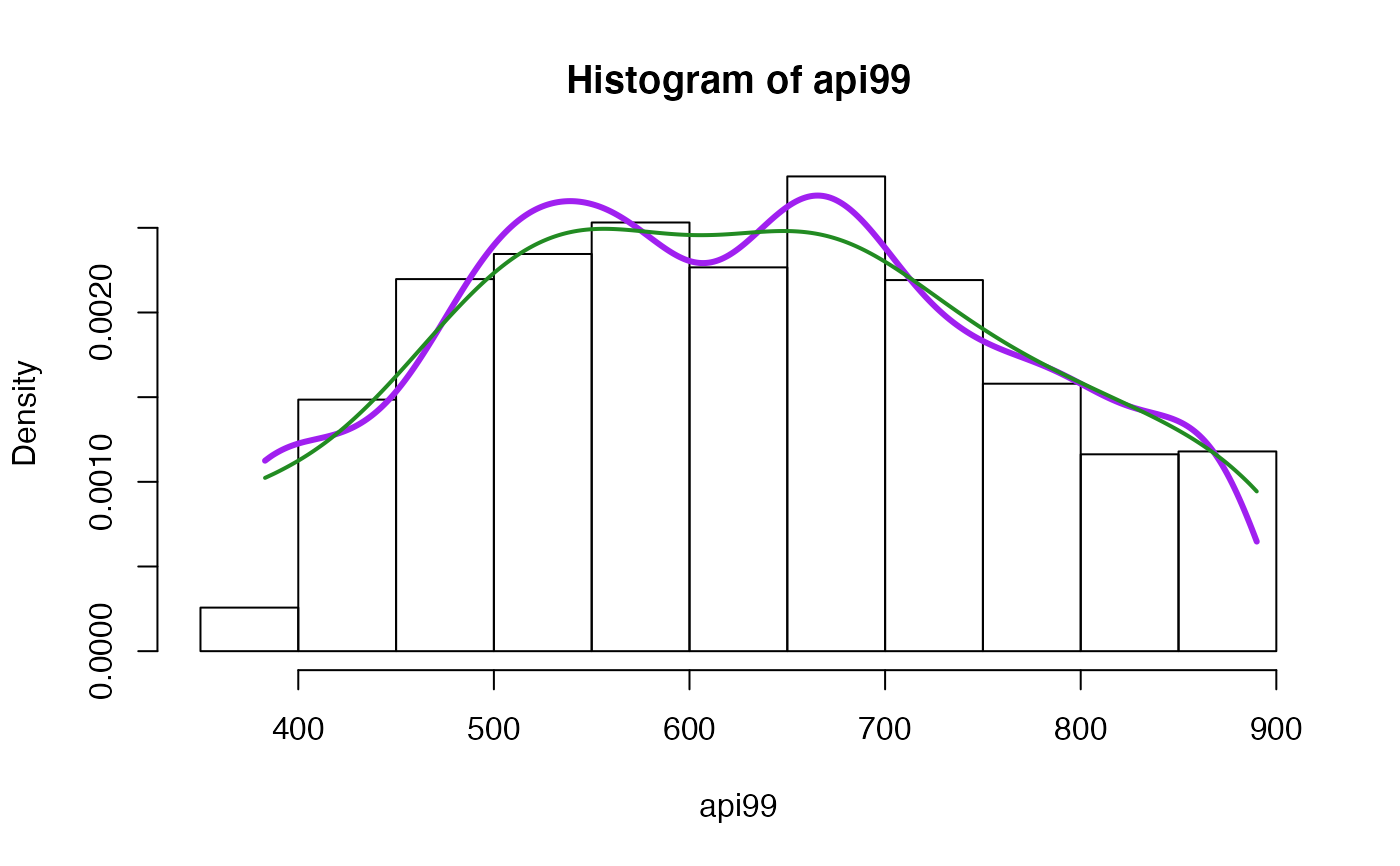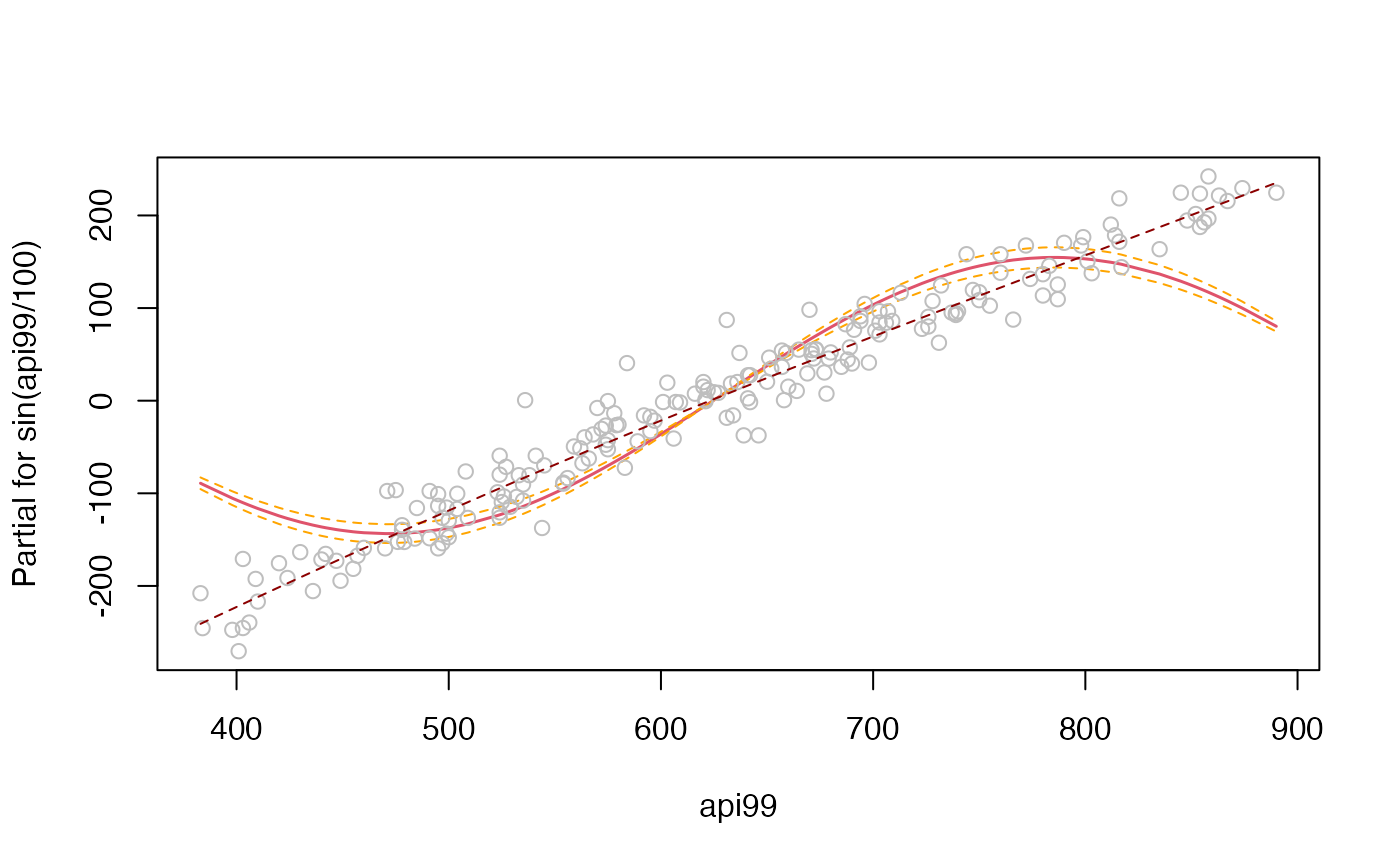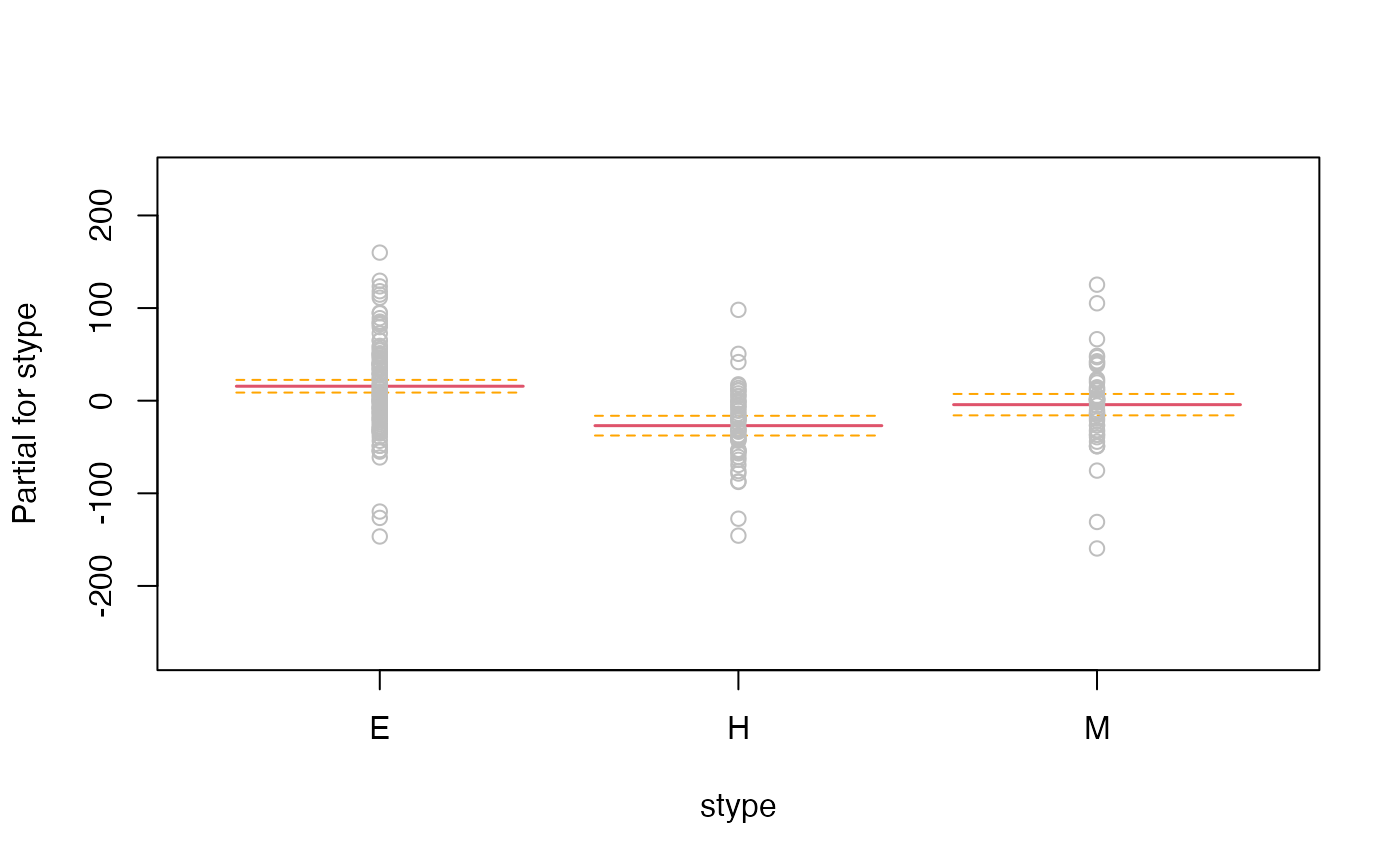Scatterplot smoothing and density estimation for probability-weighted data.

svysmooth(formula, design, ...)
# S3 method for default
svysmooth(formula, design, method = c("locpoly", "quantreg"),
bandwidth = NULL, quantile, df = 4, ...)
# S3 method for svysmooth
plot(x, which=NULL, type="l", xlabs=NULL, ylab=NULL,...)
# S3 method for svysmooth
lines(x,which=NULL,...)
make.panel.svysmooth(design,bandwidth=NULL)

## Arguments

formula

One-sided formula for density estimation, two-sided for smoothing

design

Survey design object

method

local polynomial smoothing for the mean or regression splines for quantiles

bandwidth

Smoothing bandwidth for "locpoly" or NULL for automatic choice

quantile

quantile to be estimated for "quantreg"

df

Degrees of freedom for "quantreg"

which

Which plots to show (default is all)

type

as for plot

xlabs

Optional vector of x-axis labels

ylab

Optional y-axis label

...

More arguments

x

Object of class svysmooth

## Details

svysmooth does one-dimensional smoothing. If formula has multiple predictor variables a separate one-dimensional smooth is performed for each one.

For method="locpoly" the extra arguments are passed to locpoly from the KernSmooth package, for method="quantreg" they are passed to rq from the quantreg package. The automatic choice of bandwidth for method="locpoly" uses the default settings for dpik and dpill in the KernSmooth package.

make.panel.svysmooth() makes a function that plots points and draws a weighted smooth curve through them, a weighted replacement for panel.smooth that can be passed to functions such as termplot or plot.lm. The resulting function has a span argument that will set the bandwidth; if this is not specified the automatic choice will be used.

## Value

An object of class svysmooth, a list of lists, each with x and y components.

svyhist for histograms

## Examples

 data(api)
dstrat<-svydesign(id=~1,strata=~stype, weights=~pw, data=apistrat, fpc=~fpc)

smth<-svysmooth(api00~api99+ell,dstrat)
dens<-svysmooth(~api99, dstrat,bandwidth=30)
dens1<-svysmooth(~api99, dstrat)
qsmth<-svysmooth(api00~ell,dstrat, quantile=0.75, df=3,method="quantreg")

plot(smth)plot(smth, which="ell",lty=2,ylim=c(500,900))
lines(qsmth, col="red")svyhist(~api99,design=dstrat)
lines(dens,col="purple",lwd=3)
lines(dens1, col="forestgreen",lwd=2)m<-svyglm(api00~sin(api99/100)+stype, design=dstrat)
termplot(m, data=model.frame(dstrat), partial.resid=TRUE, se=TRUE,
smooth=make.panel.svysmooth(dstrat))